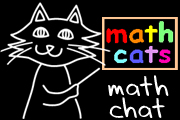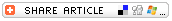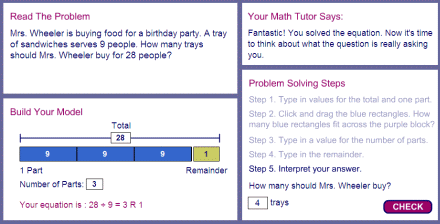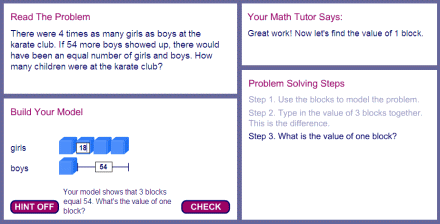## Search form# Math Problem Solving With Pictures

## By Wendy PettiPicturing a problem often is the key to helping students understand the problem and identify a solution.

We teach students many problem-solving strategies, but probably the most powerful and flexible problem-solving strategy is, "Make a picture or diagram." Picturing a problem often is the key to helping students understand the problem and identify a solution. Pictures or diagrams also can serve as prompts to help students keep track of what they need to find out in multi-step problems.

## BAR MODELS: REPRESENTING A WHOLE PROBLEM WITH A FEW SIMPLE IMAGES

Many students try to picture a problem by drawing all the details of the problem. Have you had students laboriously illustrate problems like this? Does the answer tend to get lost in the details?We can give students, instead, an approach using much simpler images for understanding the information in a problem. We might set up and solve a similar problem like this:The use of bar models is one of the visualization strategies used in the highly effective Singapore Math program. Students learn to represent simple and multi-step word problems by drawing bars to indicate how the known elements of the problem relate to one another, and then place one or more question marks to indicate what they need to find out. Bar models are a simple and powerful way to represent part-whole and whole-part relationships and to represent problems dealing with ratios. Bar modeling helps make abstract problems more concrete and facilitates algebraic reasoning in students of all ages.

Some teachers wonder if they can introduce students to bar modeling without using the full Singapore Math program. It is feasible, although many using the full program advise against it. A "taste" of bar modeling is probably not very effective; part of the power of bar modeling in the Singapore Math program is the structure and sequencing of the lessons. If you'd like to give it a try and introduce your students to the power of visual models in bar form, check out one or more of the books on the resource list at the end of this article and also use the visualization tools on the Thinking Blocks site.

Thinking Blocks is a kid-friendly interactive problem-solving resource for elementary and middle school students and their teachers. Through videos, interactive tutorials, and guided practice problems with step-by-step coaching on the sidelines, students learn to visualize and solve many types of multi-step word problems using movable blocks bearing a strong resemblance to bar models. The site uses a different visual model to help students understand how to set up a multi-step "working backwards" problem. Teachers also can model their own word problems with bars and blocks in the "model it" area.

Â

Here are a few screenshots midway through problems:Notice the input from the Math Tutor and the guided Problem Solving Steps. If a student leaves something blank or types a wrong number, the Math Tutor will provide gentle coaching. In the model area, the bars are provided at the outset, and the student must drag them into position. Question marks are used to indicate what is unknown. The arrangement and labeling of the bars and lines help students understand what they know and what they need to find out.This second problem is modeled with blocks rather than bars. (The blocks appear one at a time as each is dragged into position.) Without a visual model, many students would have a difficult time knowing how to get started in solving a problem of this type. A hint is available below the blocks in some problems; a "math tutor" responds to the student's latest action; and the student is prompted for the next step. After the student calculates that one block represents 18 children, it will be clear that the total number of children is 5 x 18.This problem is modeled with a graphic organizer utilizing arrows, rather than thinking blocks. The student must determine which operations to use and in what sequence. After setting up the problem, the student works backwards (64-19 = 45), and then calculates 87-45 to find the number of nickels used for the cookie.Could the same problem be represented with thinking blocks or bars? Here is one possible set-up:Again, after setting up the problem, we need to work backwards, solving 64 19 to find the first unknown (45) and then subtracting 45 from 87 to find the number of nickels for the cookie.

## BAR MODELS AS A TRANSITION TO ALGEBRA

Let's take a look at how a visual model can function as a bridge to algebra while taking the guesswork out of a typical "guess and check" problem: "What 2 numbers have a sum of 20 and a difference of 8?"There is more than one way to set up a problem of this type -- the next figure more closely resembles the Singapore bar modelbut the first arrangement, by including 2 of the smaller number in the model, allows us to see that two of the smaller number, added to the difference (8), will give us the sum of the two numbers (20). After the model is set up, it functions as a bridge to algebra. A blue block can be labeled b, and from there, we can write equations to express what is shown in the model and solve for a.

## ON BEYOND BAR MODELS

The bar model can be a useful tool in the problem-solving process, but we also want students to be able to express an answer in a clear written sentence and, over time, to:

• show problem-solving steps in the form of number sentences, and/or
• give a clear step-by-step explanation of the student's thinking.

Students and teachers need to appreciate that there can be a number of ways to visualize a problem, and a number of ways to solve a problem non-visually. Some students might benefit from visualization more than others.

Sometimes students resist using visual models when a solution is readily apparent to them. It might be helpful to designate certain class periods as times for learning to use a new tool; a time when students practice using the tool on very simple problems even though they don't really need it. In that way, they become comfortable with how the tool works, and are ready to apply it to more challenging problems.

Other class periods can be designated as "choose your own approach" problem-solving sessions, during which students might opt to represent problems with manipulatives, other pictorial representations, algebraic notation, or mental math. Fraction problems often are represented with pies or rectangular slices; area and perimeter problems are often represented with geometric diagrams. Some problems are more easily solved using tables or organized lists.

We want to give students a full bag of math tools, not just one! But the bar model is a tool thats finding its way into more and more students' math tool bags. Its a tool that will serve every student well.